ISSN: 1070-4698

# Vol. 64

Latest Volume
All Volumes
All Issues

2006-08-29 PIER Vol. 64, 317-333, 2006. doi:10.2528/PIER06062202

###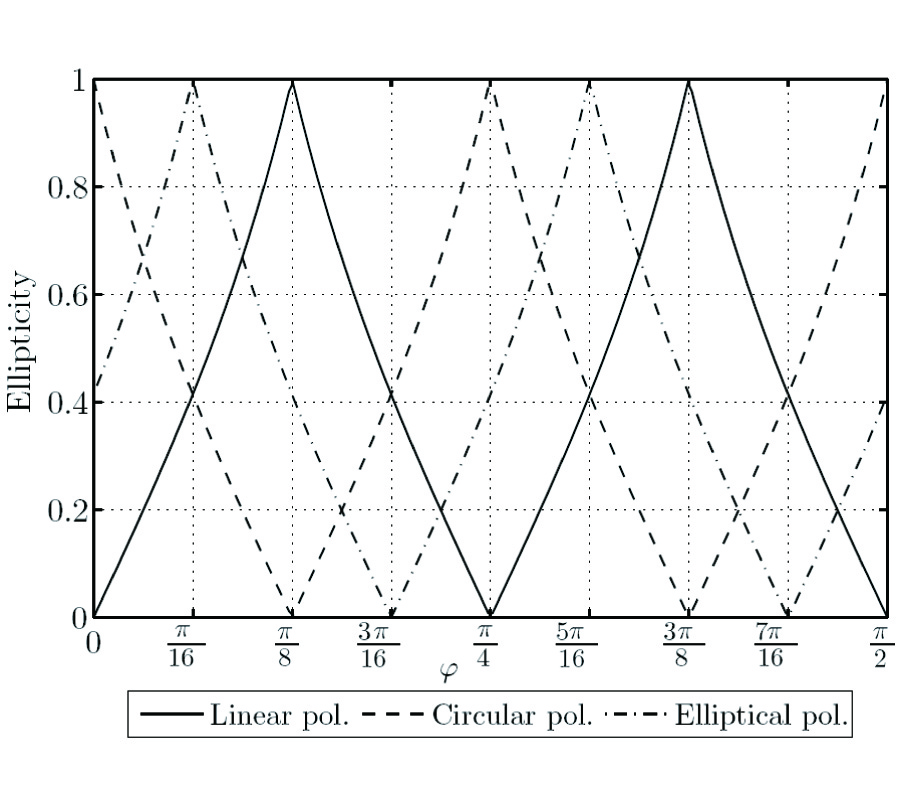Realization of Generalized Soft-and-Hard Boundary

Ilari Hanninen, Ismo Veikko Lindell, and Ari Sihvola

The classical soft-and-hard surface boundary conditions have previously been generalized to the form a Â· E = 0 and b Â· H = 0 where a and b are two complex vectors tangential to the boundary.A realization for such a boundary is studied in terms of a slab of special wave-guiding anisotropic material. It is shown that analytic expressions can be found for the material parameters and thickness of the slab as functions of the complex vectors a and b. Application of a generalized soft-and-hard boundary as a polarization transformer is studied in detail.

2006-08-29 PIER Vol. 64, 293-316, 2006. doi:10.2528/PIER06071401

###A Combination of Up- and Down-Going Floquet Modal Functions Used to Describe the Field Inside Grooves of a Deep Grating

Michitoshi Ohtsu, Yoichi Okuno, Akira Matsushima, and Taikei Suyama

An effective computational method based on a conventional modal-expansion approach is presented for solving the problem of diffraction by a deep grating. The groove depth can be the same as or a little more than the grating period. The material can be a perfect conductor, a dielectric, or a metal. The method is based on Yasuura's modal expansion, which is known as a least-squares boundary residual method or a modified Rayleigh method. The feature of the present method is that: (1) The semi-infinite region U over the grating surface is divided into an upper half plane U0 and a groove region UG by a fictitious boundary (a horizontal line); (2) The latter is further divided into shallow horizontal layers U1, U2, Â·Â·Â·, UQ again by fictitious boundaries; (3) An approximate solution in U0 is defined in a usual manner, i.e., a finite summation of up-going Floquet modal functions with unknown coefficients, while the solutions in Uq (q = 1, 2, Â·Â·Â·, Q) include not only the up-going but also the down-going modal functions; (4) If the grating is made of a dielectric or a metal, the semi-infinite region L below the surface is partitioned similarly into L0, L1, Â·Â·Â·, LQ, and approximate solutions are defined in each region; (5) A huge-sized least squares problem that appears in finding the modal coefficients is solved by the QR decomposition accompanied by sequential accumulation. The method of solution for a grating made of a perfect conductor is described in the text. The method for dielectric gratings can be found in an appendix. Numerical examples include the results for perfectly conducting and dielectric gratings.

2006-08-29 PIER Vol. 64, 279-292, 2006. doi:10.2528/PIER06070701

###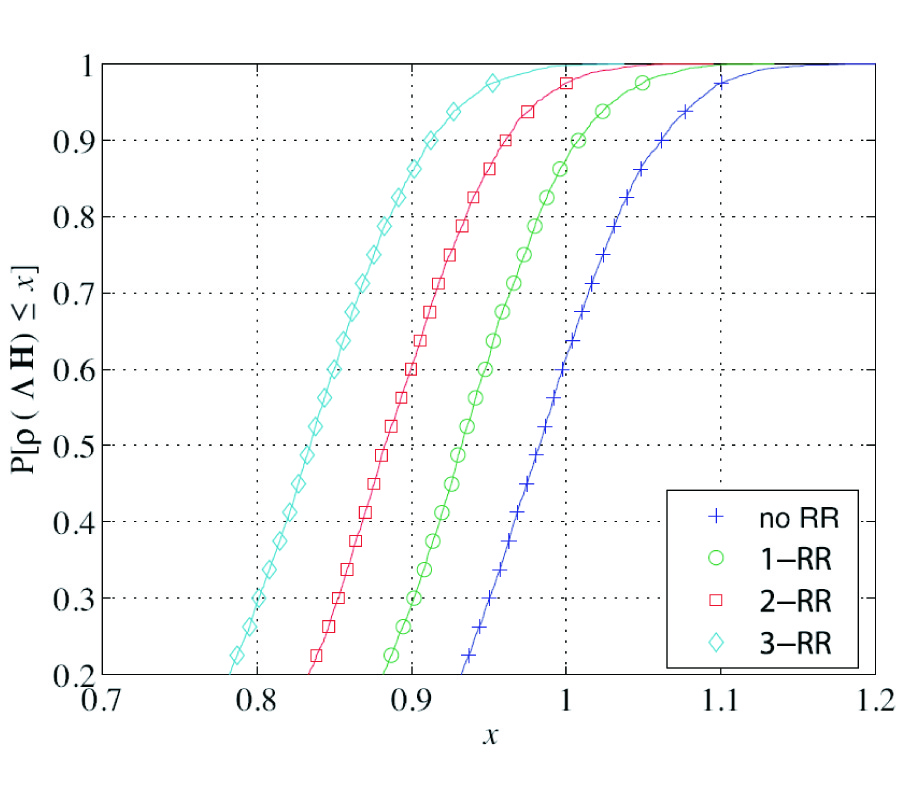Multi-Class Optical-CDMA Network Using Optical Power Control

Naser Tarhuni, Mohammed Elmusrati, and Timo Korhonenn

In this paper we use optical power control to support multirate transmission over temporal optical CDMA networks. We apply the centralized power control algorithm to set the transmit power of the users' optical sources in order to satisfy a given target QoS. In addition, optical amplifiers are included to enhance the overall system performance while the Amplified Spontaneous Emission (ASE) is considered as the main noise source. The objective function defined as the sum of the transmitted optical power from all nodes is minimized subject to a signal-to-interference (SIR) constraint. Moreover, the network feasibility, defined as the ability to evaluate a power vector that satisfy the target SIR, is discussed in terms of the spectral radius of the network interference matrix. Next, the spectral radius of the network interference matrix is investigated and modeled as a truncated Gaussian distribution. Last, a rate reduction algorithm, categorized in terms of the number of nodes involved in the process of rate reduction, is proposed to increase the network feasibility. As more nodes are added to the rate reduction campaign, the network feasibility is significantly enhanced. For typical network parameters we find by simulating 104 random network realizations that a threenode rate reduction results in 99% network feasibility.

2006-08-29 PIER Vol. 64, 257-278, 2006. doi:10.2528/PIER06050801

###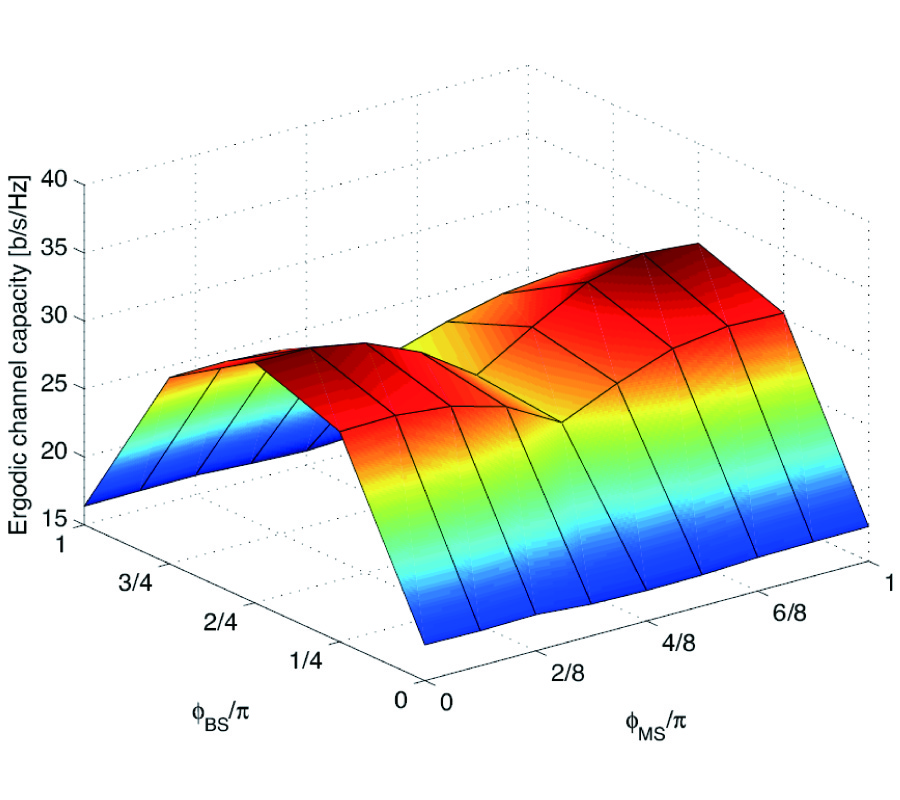Effect of Antenna Array Geometry and Ula Azimuthal Orientation on MIMO Channel Properties in Urban City Street Grid

Abdulla Abouda, Hassan El-Sallabi, and S. Häggman

Propagation environment and antenna array configuration have significant effect on spatial correlation properties of multipleinput multiple-output (MIMO) wireless communications channels. In this paper the effect of different antenna array geometries on MIMO channel properties is investigated in urban city street grid propagation environment. Four antenna array geometries with the same number of antenna elements and fixed inter-element spacing are considered, namely, uniform linear array (ULA), uniform circular array (UCA), uniform rectangular array (URA) and uniform cubic array (UCuA). The effect of ULA orientation in azimuthal plane on MIMO channel ergodic capacity is also investigated. Varying orientation angle from 0 to Ï€ at the two communication ends is considered. The investigation is carried out based on three dimensional (3D) spatial multi-ray realistic propagation channel model covering different propagation types. It is shown that the antenna array geometry have significant impact on MIMO channel properties. Under different propagation scenarios the ULA shows superiority to the other considered geometries in terms of the ergodic channel capacity and number of spatial parallel channels. However, this superiority depends largely on the array azimuthal orientation.

2006-08-29 PIER Vol. 64, 239-255, 2006. doi:10.2528/PIER06080201

###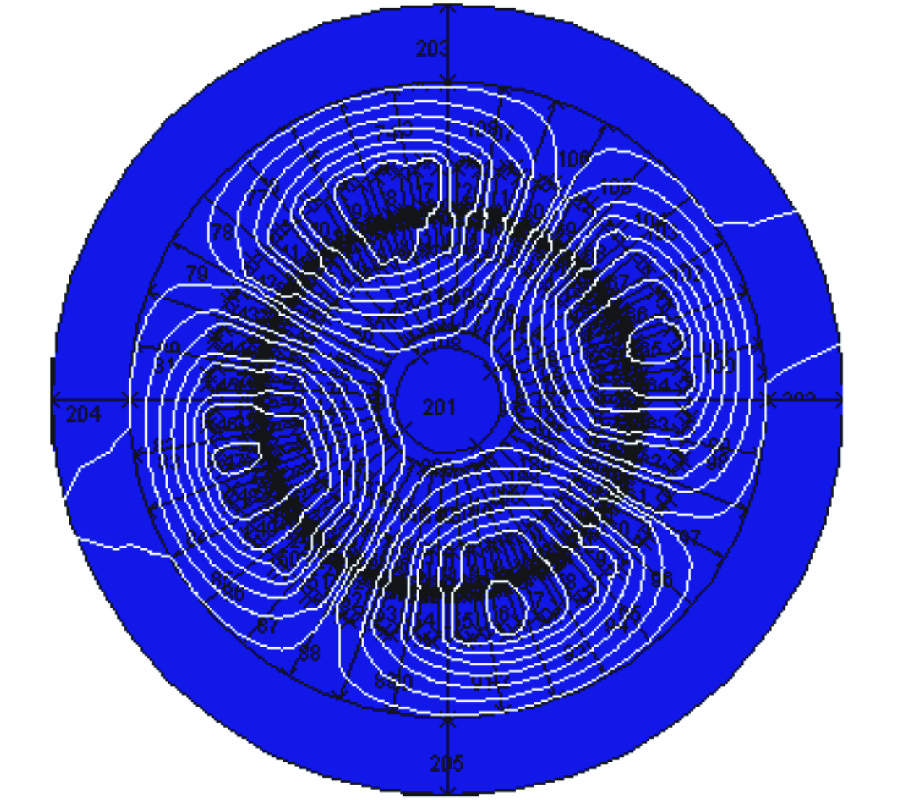Mixed Fault Diagnosis in Three-Phase Squirrel-Cage Induction Motor Using Analysis of Air-Gap Magnetic Field

In this paper fault diagnosis of induction motor under mixed fault is carried out using precise analysis of the air-gap magnetic field based on time stepping finite element method. By feeding voltage instead of current density to the finite element computation part, the drawbacks of the application of finite element method in fault diagnosis of induction motor are overcome. Normally static eccentricity and broken rotor bars faults have been individually diagnosed in the published papers. Here diagnosis of the mixed fault,including static eccentricity and broken rotor bars,is introduced which is considered as a novel part of the present work. Precise analysis of magnetic field in the air-gap of a faulty induction motor is carried and performance of the motor is predicted. Taking into account the magnetic core saturation is another advantage of the present work. This is required in the transient analysis of a faulty motor.

2006-08-29 PIER Vol. 64, 219-238, 2006. doi:10.2528/PIER06071902

###Analysis of Linear Tapered Waveguide by Two Approaches

Santanu Dwari, Ajay Chakraborty, and Subrata Sanyal

This paper presents the analysis of linear tapered waveguide. Voltage-standing-wave-ratio (VSWR) is obtained from transmission matrix of the taper waveguide. Taper section is divided into number of section having uniform length. Transmission matrix of taper waveguide is found by multiplication of transmission matrix of each section. Transmission matrix of each section is obtained as the product of three matrices. One is of the initial length of transmission line,second one is due to discontinuity and third one is of the final length of transmission line. Transmission matrix of discontinuity is obtained by two methods. One is by equivalent circuit of step discontinuity and another is by moment method. The results are seen to be in good agreement with [1,2] and .

2006-08-29 PIER Vol. 64, 205-218, 2006. doi:10.2528/PIER06071104

###Novel Broadband Terahertz Negative Refractive Index Metamaterials: Analysis and Experiment

Nantakan Wongkasem, Alkim Akyurtlu, Jin Li, Adam Tibolt, Zeng Kang, and William Goodhue

Broadband planar and non-planar negative refractive index (NRI) metamaterial (MTM) designs consisting of a periodically arranged split ring resonator and wire structures are developed in the terahertz (THz) frequency regime using the Finite-Difference Time- Domain (FDTD) method. The novel MTM designs generate a broad negative index of refraction (NIR) passband approximately two and a half times higher than those of the conventional SRR/wire structures, by using the same dimensions. Numerical simulations of wedgeand triangle-shaped metamaterials are used to prove the negative refractive index of the models. The fabricated MTMs exhibit passband characteristics which are in good agreement with the model results. The parametric studies of correlated factors further support these outcomes.

2006-08-29 PIER Vol. 64, 191-204, 2006. doi:10.2528/PIER06071101

###Modal Analysis and Dispersion Curves of a New Unconventional Bragg Waveguide Using a Very Simple Method

Vivek Singh, Yogendra Kumar Prajapati, and Jai Prakash Saini

A theoretical modal dispersion study of a new unconventional Bragg waveguide having hypocycloidal core cross-section and surrounded by Bragg cladding layers is presented using a very simple boundary matching technique . An attempt has been made to determine how the modal characteristics of a standard Bragg fiber change as its circular shape is changed to the hypocycloidal shape. It is seen that in the case of a hypocycloidal Bragg waveguide single mode guidance is possible when V â‰¤ 10.0 where V is the normalized frequency parameter.

2006-08-28 PIER Vol. 64, 167-189, 2006. doi:10.2528/PIER06062102

###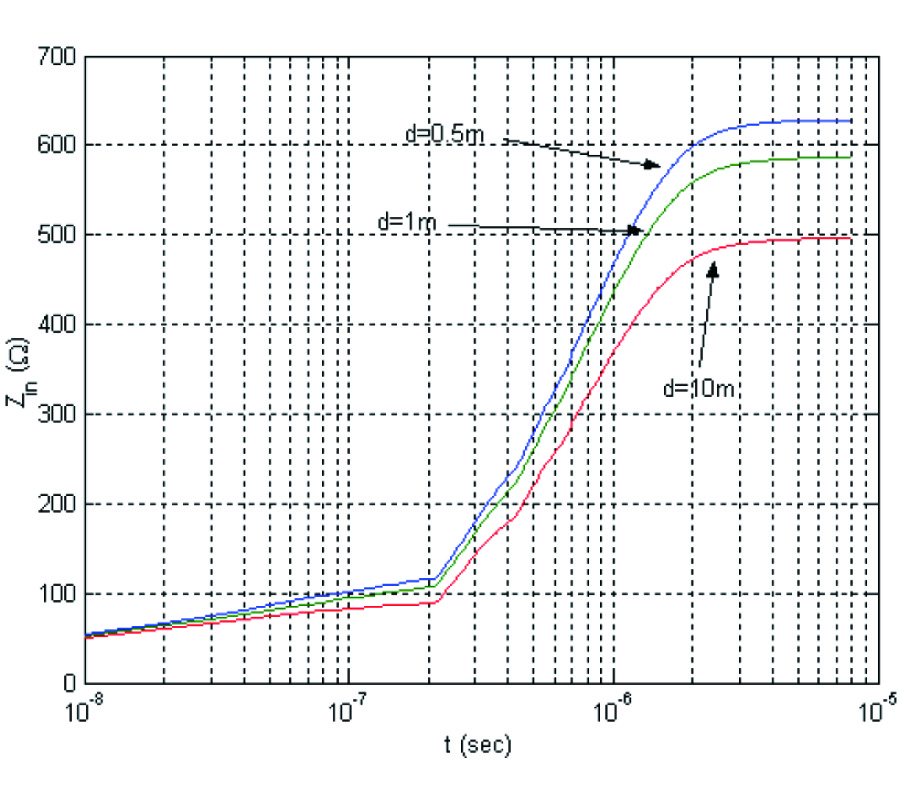Wire Antenna Model for Transient Analysis of Simple Grounding Systems, Part II: the Horizontal Grounding Electrode

Dragan Poljak and Vicko Doric

In the Part I of this work general introduction to the transient impedance assessment of simple grounding systems has been presented. Part I also deals with the analysis of the vertical grounding electrode, while this paper analyses a more demanding case of the horizontal electrode.The mathematical model is based on the thin wire antenna theory featuring the Pocklington integro-differential equation. The Pocklington equation is solved using the Galerkin-Bubnov indirect Boundary Element Method. The details are available in Appendix. The formulation of the problem is posed in the frequency domain, while the corresponding transient response of the grounding system is obtained by means of the inverse Fourier transform. Some illustrative numerical results are shown throughout this work.

2006-08-28 PIER Vol. 64, 149-166, 2006. doi:10.2528/PIER06062101

###Wire Antenna Model for Transient Analysis of Simple Grounding Systems, Part I: the Vertical Grounding Electrode

Dragan Poljak and Vicko Doric

The paper deals with the transient impedance calculation for simple grounding systems. The mathematical modelmodel is based on the thin wire antenna theory. The formulation of the problem is posed in the frequency domain, while the corresponding transient response of the grounding system is obtained by means of the inverse Fourier transform. The current distribution induced along the grounding system due to an injected current is governed by the corresponding frequency domain Pocklington integro-differential equation. The influence of a dissipative half-space is taken into account via the reflection coefficient (RC) appearing within the integral equation kernel. The principal advantage of the RC approach versus rigorous Sommerfeld integral approach is simplicity of the formulation and significantly less computational cost. The Pocklington integral equation is solved by the Galerkin Bubnov indirect boundary element procedure thus providing the current distribution flowing along the grounding system. The outline of the Galerkin Bubnov indirect boundary element method is presented in Part II of this work. Expressing the electric field in terms of the current distribution along the electrodes the feed point voltage is obtained by integrating the normal field component from infinity to the electrode surface. The frequency dependent input impedance is then obtained as a ratio of feed-point voltage and the value of the injected lightning current. The frequency response of the grounding electrode is obtained multiplying the input impedance spectrum with Fourier transform of the injected current waveform. Finally, the transient impedance of the grounding system is calculated by means of the inverse Fourier transform. The vertical and horizontal grounding electrodes, as simple grounding systems, are analyzed in this work. The Part I of this work is related to the vertical electrode, while Part II deals with a more demanding case of horizontal electrode.

2006-08-28 PIER Vol. 64, 135-148, 2006. doi:10.2528/PIER06061601

###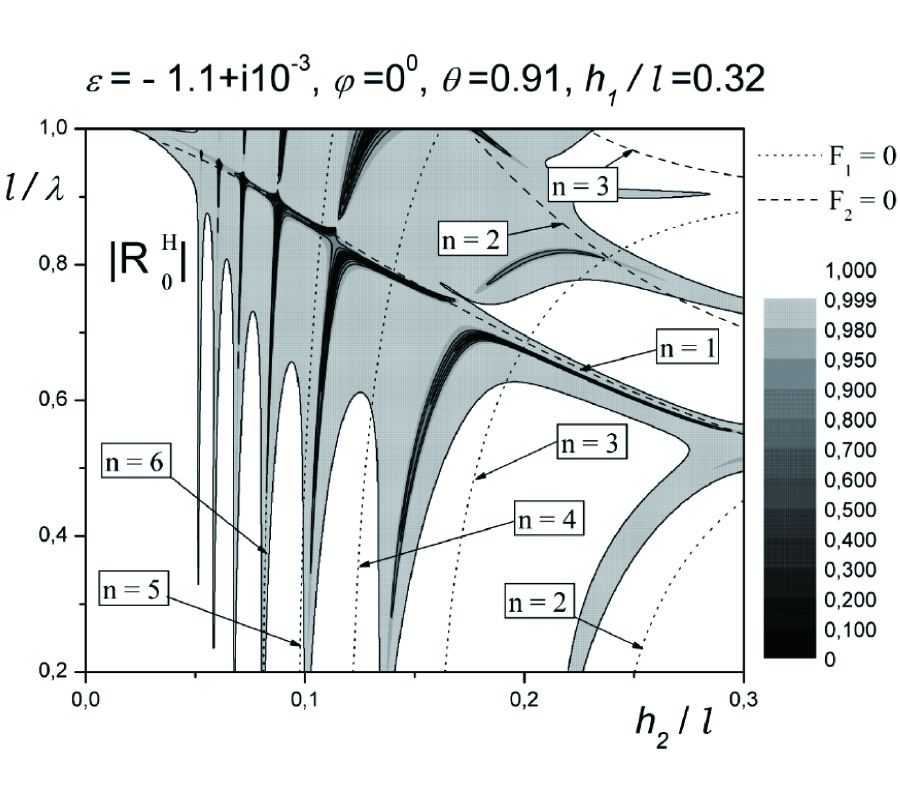Absorbing Properties of a Negative Permittivity Layer Placed on a Reflecting Grating

O. Kusayakin, Petr Melezhik, Anatoly Poyedinchuk, and Oleksander Troschylo

Theoretical results on the electromagnetic properties of such a structure as a negative-permittivity layer placed on a reflecting grating are discussed. Effect of this structure resonant absorption of the excitation electromagnetic energy is studied in a wide range of problem parameters. The sufficient conditions of the effect appearance are determined by solving the dielectric-layer eigenoscillation problem. A comparative analysis of the absorption by the negative- and the positive-permittivity structures is made to reveal the characteristic features of the effect. Phenomenon of the absorption resonance splitting is shown in the case of plane wave oblique incidence on the structure. The E-polarized plane wave diffraction is considered to find out essential difference of the resonant absorption in the E and H cases.

2006-08-28 PIER Vol. 64, 117-134, 2006. doi:10.2528/PIER06061302

### On Independence, Completeness of Maxwell's Equations and Uniqueness Theorems in Electromagnetics

Xingling Zhou

In this paper, the independence, completeness of Maxwell's equations and uniqueness theorems in electromagnetics are reviewed. It is shown that the four Maxwell's equations are independent and complete. A complete uniqueness theorem is proposed and proven for the first time by pointing out logic mistakes in the existing proof and presenting a truth table. Therefore, electrostatics and magnetostatics can be reduced from dynamical electromagnetics in all aspects including not only the equations as subsets of Maxwell's equations but also the corresponding uniqueness theorems. It is concluded that the axiomatic system of electromagnetic theory must consist of all four Maxwell's equations.

2006-08-28 PIER Vol. 64, 99-116, 2006. doi:10.2528/PIER06052404

###Broadband Scattering Data Interpolation Based on a Relaxed Adaptive Feature Extraction Algorighm

Anna Raynal, John Moore, and Hao Ling

A method using a relaxed adaptive feature extraction algorithm is investigated to interpolate broadband, high-frequency scattering from sparse, undersampled data. First, the adaptive feature extraction is extended to include a frequency-dependent scattering center model in order to better-describe broadband scattering physics from a complex target per the geometrical theory of diffraction. Second, the parameterization of the model is relaxed for more accurate extractions and a sparser model representation using fewer samples. Comparative results are presented for the relaxed versus the non-relaxed adaptive feature extraction algorithm for hypothetical examples and a numerically-solved ogive body of revolution. The relaxed algorithm is more computationally expensive, but results in significantly improved performance. The technique enhances adaptive feature extraction performance for broadband interpolation.

2006-08-28 PIER Vol. 64, 81-98, 2006. doi:10.2528/PIER06060602

###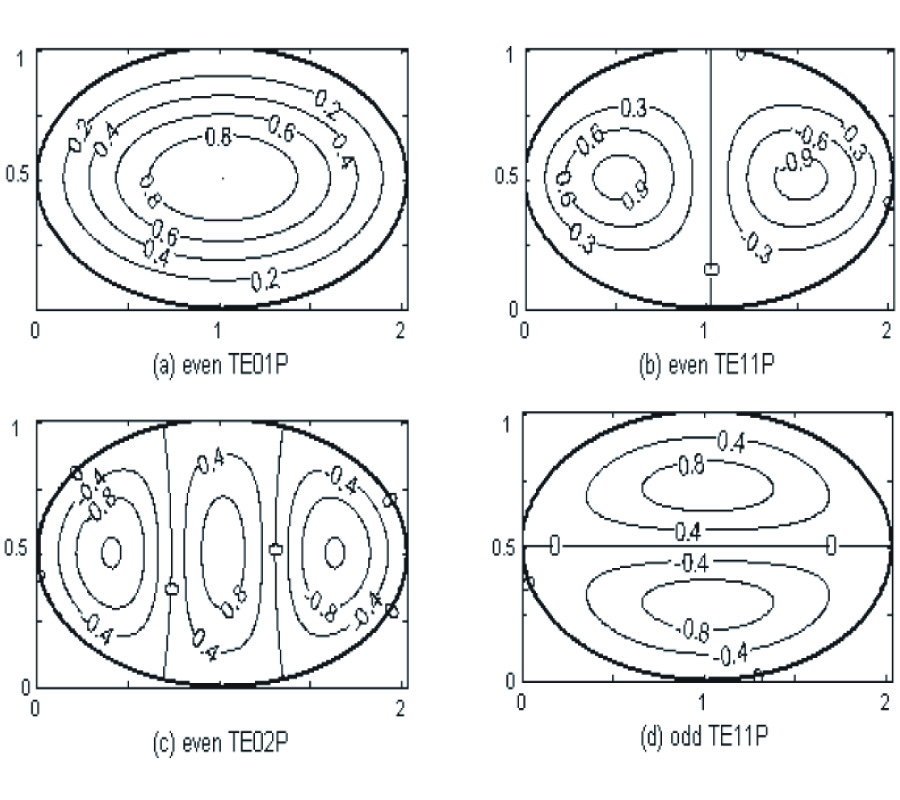Resonance Frequencies and Far Field Patterns of Elliptical Dielectric Resonator Antenna: Analytical Approach

A. Tadjalli, Abdel Sebak, and Tayeb Denidni

In this paper the classical boundary value approach employing the separation of variable technique is used to analyze the properties of elliptic dielectric resonator antennas. In this approach, the fields inside the resonator are expanded in terms of Mathieu and modified Mathieu functions. Numerical results are given for resonant frequencies of different modes as well for fields distribution inside the resonator. Series Green's functions are used to calculate the fields inside the cavity and also far field patterns for a given feed. Using the Green's function method provides more accurate results compared to a pure cavity model technique. The analysis and design are verified through numerical simulations. A parametric study has been performed to show effects of the elliptical dielectric resonator antennas (EDRAs) parameters on far field patterns.

2006-08-28 PIER Vol. 64, 69-80, 2006. doi:10.2528/PIER06071301

###FDTD Analysis of an Anisotropically Coated Missile

Zhuqian Gong and Guo-Qiang Zhu

In this paper, the finite-difference time-domain (FDTD) method is applied to calculate the radar cross-section (RCS) of anisotropic coated missiles. We present a FDTD algorithm for cylindrical coordinates and derive the necessary extension to the FDTD equations for a general three-dimensional anisotropic scatterer. A new approach is proposed to obtain magnitude and phase information from the FDTD data. Computed results for two selected examples are compared with those obtained by eigenfunction expansion techniques and the moment method (MM) to demonstrate the validity of the new approach with very good agreement. The study of the bistatic radar cross-section for a missile demonstrates that the anisotropic material coating around it can effectively reduce its RCS.

2006-08-28 PIER Vol. 64, 53-67, 2006. doi:10.2528/PIER06052403

###Electromagetic Pulse Diffraction by a Moving Half-Plane

This paper is concerned with the scattering of an electromagnetic (EM) pulse by a perfectly conducting half-plane, moving in a free space. It is assumed that the source signal is a plane wave pulse with its envelope described by a Dirac delta function. The representation for the total field is found, and physical interpretation of the solution is given. This representation, valid for all screen velocities, is then reduced to the case of moderate and low velocities, important for practical applications.

2006-08-28 PIER Vol. 64, 43-51, 2006. doi:10.2528/PIER06061301

###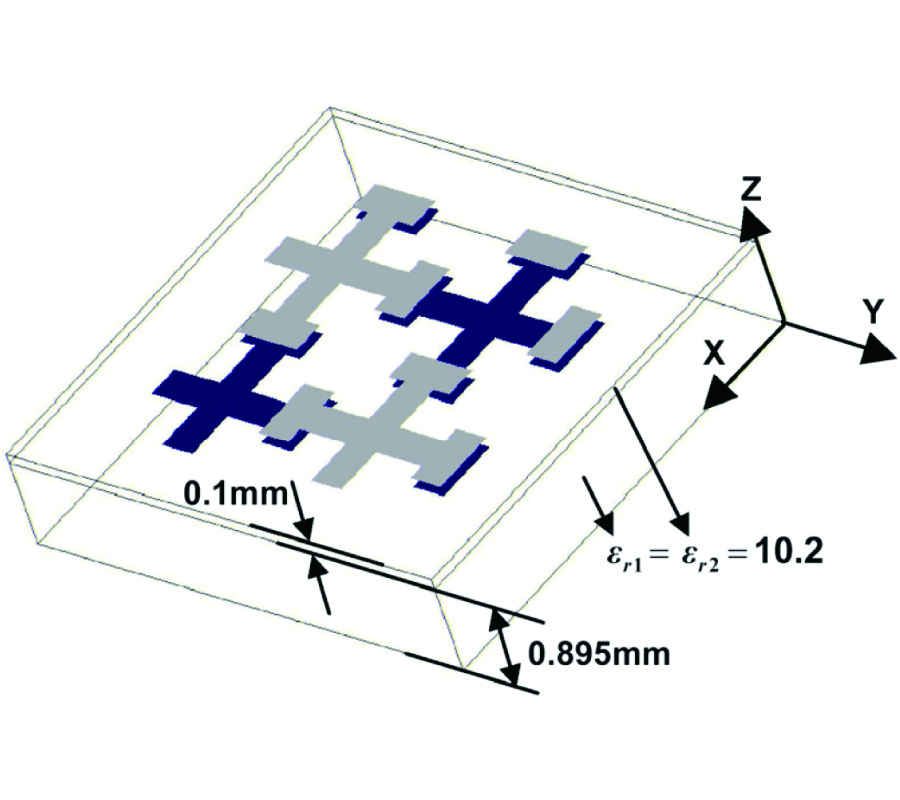A Novel AMC with Little Sensitivity to the Angle of Incidence Using 2-Layer Jerusalem Cross FSS

A novel artificial magnetic conductor (AMC), i.e., highimpedance surface (HIS), is proposed. The structure, which utilizes the well know Jerusalem Cross Frequency Selective Surface (JC-FSS), is a novel 3D AMC. It offers stable resonance frequency with respect to the incidence angle of both TE and TM-polarized plane waves, very compact size and acceptable bandwidth.

2006-08-28 PIER Vol. 64, 23-42, 2006. doi:10.2528/PIER06062401

###Diversity Techniques with Parallel Dipole Antennas: Radiation Pattern Analysis

Ali Khaleghi

Two parallel dipoles are assessed for antenna diversity. The three-dimensional radiation pattern is considered for signals correlation coefficient. The pattern analysis reveals that, depending on dipole spacing, three types of diversity techniques are generated: space, amplitude-pattern and phase-pattern diversity. The weighting of each technique in signals correlation coefficient mitigation is investigated. The results show that for closely spaced dipoles, the generated phasepattern diversity is the most dominant factor which greatly reduces the signals correlation coefficient. The diversity configuration is measured in a rich scattering environment. Results include signals correlation coefficient, diversity gain for selection combining and maximum ratio combining, effective diversity gain and antenna radiation efficiency can be demonstrated. We show that in rich multipath channel the minimum spatial distance, for effective diversity gain performance, is reduced from 0.5Î» for uncoupled dipoles to 0.15Î» for coupled dipoles.

2006-08-28 PIER Vol. 64, 1-21, 2006. doi:10.2528/PIER06060802

###Numerical Analysis of Optical Waveguides Based on Periodic Fourier Transform

Koki Watanabe and Kousuke Kuto

Periodic Fourier transform is formally introduced to analyses of the electromagnetic wave propagation in optical waveguides. The transform make the field components periodic and they are then expanded in Fourier series without introducing an approximation of artificial periodic boundary. The proposed formulation is applied to two-dimensional slab waveguide structures, and the numerical results evaluate the validity and show some properties of convergence.